United States Department of LaborThe .gov means it's official.
Federal government websites often end in .gov or .mil. Before sharing sensitive information, make sure you're on a federal government site.The site is secure.
The https:// ensures that you are connecting to the official website and that any information you provide is encrypted and transmitted securely.

Producer Price Indexes

# Variance estimates for price changes in the Producer Price Index

The Producer Price Index (PPI) program at the Bureau of Labor Statistics (BLS) began publishing variance estimates in 2016 based on data for 2015. PPI intends to publish variance estimates on an annual basis. This document outlines the uses for variance statistics and provides a description of PPI variance estimation methodology.

### Survey error

Survey error can be classified into sampling error and nonsampling error. Sampling error results when a parameter is calculated on the basis of a sample instead of the full population. The PPI program collects price information for a sample of more than 100,000 goods and services on a monthly basis. These transaction prices are used to construct the broad range of PPIs. PPI variance estimates measure the sampling error in the estimate of the percent change in an index. Sampling error reflects the difference between a sample estimate of change and the true population value. Variance statistics measure the precision of a sample estimate.

Nonsampling error occurs in many forms, but whatever the form, nonsampling error does not affect the spread of the sample estimates. Nonsampling error can, however, cause the estimate to be consistently higher (or lower) than the true population estimate. When this occurs, it is called the bias of a sample estimate. One example of sample estimate bias is nonresponse error. Each month, there are establishments in the PPI sample for which no price data are received. If the subset of the sample that fails to provide price update information differs consistently in some way from the subset that does respond, bias is created in the estimate. Another type of nonsampling error occurs when the frame from which the sample was drawn does not properly represent the population. Still other nonsampling errors occur during the collection of data. For example, if instructions do not provide clear directions regarding what is to be collected, BLS economists may fail to communicate their requests clearly or may make errors in recording the data they collect. If any of these errors appear on a consistent basis, a bias is created in the estimate. The PPI program, along with other programs at BLS, analyzes estimates for the presence of bias and mitigates nonsampling error by conducting dedicated research into the population of interest, by clearly documenting data collection procedures, and by performing quality checks throughout the collection process.

A sample estimate with high variability, but no bias, is less precise, but has an expected value equal to that of the true population value. By contrast, a sample estimate with low variability, but a large bias, may prove consistent with other sample estimates from the population sampled, but does not have an expected value equal to the population value. An ideal sample estimate has a small variance and no bias.

### Description of data

Each month, the PPI program calculates 1-month and 12-month percent changes and their associated variance estimates. The values that constitute the annual PPI publication of variance statistics include the median of the absolute values of the percent change as a reference statistic, as well as the corresponding median standard error as the measure of variance, both based on 1-month and 12-month percent changes for the calendar year.

A commonly used measure of sampling variability is the standard error (SE), which is the square root of the variance estimate. The SE estimates the sample distribution, or spread of the sample estimates, for comparison with the true population value. The SE is used to calculate a confidence interval around the sample percent change estimate. To calculate a 95-percent confidence interval, data users commonly add and subtract 2 times the SE value from its corresponding percent change estimate to obtain a range of values that likely contains the true population percent change. If this range includes zero, then the percent change is not significantly different from zero.

In analyzing variance in the PPI, the median of the absolute value of the percent change is the reference statistic that best represents the magnitude of the percent change in an index. If, instead, the median value of the signed percent changes over a calendar year were used for an index that exhibits bidirectional volatility, then the resulting median value might be small while the discrete values themselves would include a number of large increases and decreases. The median value of 12 months of signed percent changes would then fail to correspond with the median standard error for the calendar year, because the individual, larger index percent changes likely have larger standard errors associated with them and standard errors are always positive. For example, an index that increased by 5 percent 6 months of the year and decreased by 5 percent the other 6 months of the year could have an SE of 1 percent for each month. The median percent change would then be zero, but the median SE would be 1 percent. The linkage between these two values would break down because of the bidirectional volatility of the index. In contrast, employing the median of the absolute values of the percent change provides a reference statistic of 5 percent, which is a more appropriate reference value when paired with the median SE value of 1 percent.

PPI variance estimates include data for the North American Industry Classification System (NAICS), the Final Demand-Intermediate Demand (FD-ID) system, and the unique-to-PPI, commodity-based index structure. For the NAICS-based industry structure, variance data are available for industry sector indexes; 3-, 4-, and 5-digit industry group indexes; and for 6-digit industry indexes. Six-digit industries are the basic unit for sampling and data collection in PPI. The FD-ID system is the main PPI structure for analyzing producer inflation at the aggregate level. Variance data are provided for the entire FD-ID aggregation system. Within the PPI commodity-based index structure, 6-digit commodity indexes are the detailed components of the FD-ID system. Data users commonly use 4-digit and 6-digit PPI commodity indexes to explain FD-ID index movements. Variance data are provided for 2-, 3-, 4-, and 6-digit commodity-based indexes.

### Analyzing the data

Sampling error can be affected by a number of factors, such as the size and design of the sample, as well as the variability of the population of interest. The larger the sample size, the more accurate is the representation of the population and the smaller is the deviation from the true population value. If every item in the population were selected into the sample, the sample mean and the population mean would be equal and the sample estimate would have a standard error of zero. This tendency toward equality of the sample mean and the population mean and toward a standard error of zero can be observed in the PPI: typically, the standard errors of index percent changes for more highly aggregated indexes tend to be smaller than the standard errors of percent change estimates of more detailed indexes. This is not always the case, however, because other factors also affect the standard error. In addition, standard errors typically decrease, on a relative basis, compared with their accompanying reference measures, when the analysis shifts to a 12-month basis rather than a 1-month basis.

For example, the 1-month median absolute percent change in the final demand goods index for 2016 was 0.46 percent and the accompanying SE value was 0.10 percent. Thus, two standard error values, plus and minus, provide 95-percent confidence that the true change in the median absolute 1-month change in the final demand goods index was between 0.26 percent and 0.66 percent in 2016. Looking at 12-month data for the final demand services index in 2016 reveals that the median absolute change was 1.32 percent, the SE was 0.47 percent, and the 95-percent confidence interval was between 0.38 percent and 2.26 percent. The confidence intervals for the major components of the final demand index varied in 2016, with the relative size of the confidence band for the goods component being somewhat narrower compared to the relative size of the confidence interval for the services component of final demand, which exhibited somewhat wider banding. (See table A.)

Table A. Variance estimates for selected PPI Final Demand–Intermediate Demand indexes, 2016

 Index 1-month median absolute percent change 1-month median standard error 12-month median absolute percent change 12-month median standard error Final demand .20 .11 .14 .33 Final demand, goods .46 .10 2.09 .19 Final demand, services .14 .16 1.32 .47 Final demand, construction .05 .06 .92 .21

When the calculated 95-percent confidence interval contains a value of zero, the interpretation is that there is not a significant percent change for the given statistic. For example, in 2016 the 1-month median absolute percent change for the final demand index was 0.20 percent, while the SE value was 0.11 percent. Subtracting two SE values from the 0.20 median absolute percent change results in a range of values that contains zero. This means that, at the two SE confidence level, zero is a possible outcome of the percent change, and the statement that the percent change differs from zero cannot be made. For this particular example, seven months in 2016 had a 1-month rate of change in the PPI for final demand that ranged from -0.2 percent to +0.2 percent, resulting in the median absolute percent change of 0.2. In contrast, while the 2015 SE for the final demand index also was 0.11 percent, the 1-month median absolute percent change was larger, 0.30 percent. Those results provided directional confidence at the two SE level in 2015. The SE for the aggregate index (in this case final demand) is contributed to by the various components by which it is comprised (final demand for goods, services and construction). If a component is more volatile, it will be reflected in the SE of its aggregate.

### PPI sampling

Three times each year, the PPI program updates its samples for approximately 25 to 30 industries. Industries are defined according to the North American Industry Classification System (NAICS). PPI industry samples last, on average, about 7 years, and updating samples in this manner distributes the burden of data collection over time. The decision when to select a new sample for an industry is based on a number of factors, including the age of the current sample, its level or rate of attrition, the rate at which producers enter and exit the industry, and the degree that product and service lines undergo dynamic change. Once an industry is chosen for sampling, an industry study is undertaken to identify unique characteristics of that industry and to determine whether special considerations need to be taken into account in designing the sample, initiating data collection, and requesting monthly price updates. The PPI program typically conducts initial data collection via interviews with producers that are sampled. The number of units allocated to an industry’s new sample depends on a variety of factors, including number of producers in the industry, original size of the current sample, deterioration rate, and current variance estimate.

The drawing of an industry sample takes place in two stages. The first stage is the selection of establishments, using probability-proportional-to-size sampling. The PPI program typically selects industry-based samples of establishments from the Unemployment Insurance (UI) frame—a file that lists all businesses that pay unemployment insurance. This file provides size measure data in terms of either employment or wages. However, for some industries—particularly services industries—the PPI program makes use of alternative frame sources that have more suitable size measures. The size measure of a frame serves as a proxy for establishment revenue and is used to calculate an establishment’s weight in the PPI survey. The best sampling size measure is determined on an industry-by-industry basis. At this stage, some establishments are selected with certainty, because they have a large size measure, whereas others have a probability of selection. These establishments are known as certainty and probability establishments, respectively. Although each industry is itself an explicit stratum (a nonoverlapping subgroup), information obtained during the industry study process, as well as the data available from the frame, determine whether further stratification is necessary. Examples of additional stratification variables are the industry’s geographic dispersion and size category of its producers.

The second-stage sample is drawn when BLS economists conduct initiation interviews with officials at the establishments selected in stage 1. In stage 2, the economist obtains a more accurate measure of establishment size by obtaining actual revenue information from the sample unit. Then, using a probability-based disaggregation method that identifies unique characteristics of products and transactions, the economist selects a sample of transactions of goods or services whose prices will be tracked over time. Information collected during initiation is entered into the PPI database. Price update requests are then sent to respondents on a monthly basis for the life of the sample.

Two industries with the same sample size can have very different standard errors because of the variability, or spread, of the population from which the sample is drawn. Industry samples are designed to account for diverse types of establishments that experience different kinds of price movements in a population. For example, the industry designated “offices of real estate agents and brokers” (NAICS 531210) has proportional representation of both residential and nonresidential offices and transactions. Proportionally allocating a portion of the sample to each stratum of a population, rather than relying on a random draw of the entire population, ensures better representation of the population. As mentioned previously, another key factor in the standard error of an estimate is the variability of the population itself: some industries experience very small price movements while others exhibit volatile pricing.

In certain cases, primarily PPIs for agricultural products, price data are not directly sampled by PPI staff. Instead, the data are collected from secondary sources, mainly the U.S. Department of Agriculture, but also other sources. For PPI purposes, these data are considered a census of available information, and as a result, no sampling variability exists in the percent change estimates based on them, and their standard error values are zero. These standard error values contribute to higher level aggregations, and at some point, aggregate indexes include both sampled and nonsampled data. In particular, all commodity codes under PPI group 01, Farm Products, are calculated from nonsampled data, as is the FD–ID index for Unprocessed Foodstuffs and Feedstuffs. Furthermore, the latter index constitutes a substantial portion of the aggregate index for Unprocessed Goods for Intermediate Demand.

### Calculating percent change estimates

The PPI calculates variance statistics for 1-month and 12-month percent change estimates. To understand the specific calculations, it is important to have a general understanding of how PPIs are calculated. The rest of this section provides an explanation of those calculations.

Calculating the long-term relative for items in a lowest level cell index:

The PPI calculates the long-term price relative for each item with a price that is considered good. Essentially, a good price is an item price that does not require imputation because a valid price was collected from the respondent during a given period. To calculate the long-term price relative, the PPI divides the current-period price of an item by the base-period price of that item: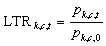,

where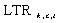is the long-term price relative of item k with a good price in cell c at time t,is the price of item k in cell c at time t, andis the price of item k in cell c in base period 0.

Calculating the cell aggregate for a lowest level cell index:

To calculate the cell aggregate, the PPI multiplies the long-term price relative of each item by the weight of the item and sums across the items in the cell: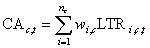Here,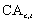is the cell aggregate for cell c at time t,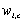is the weight of item i in cell c,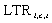is the long-term price relative of item i in cell c at time t, and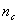is the total number of items in cell c.

Calculating the cell-level index:

To calculate the cell-level index, the PPI divides the cell aggregate for the current period by the cell aggregate for the previous period and multiplies by the cell-level index for the previous period:,

In this equation,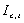is the index for cell c at time t,is the index for cell c at time t – 1,is the cell aggregate for cell c at time t, and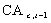is the cell aggregate for cell c at time t – 1.

Lower level indexes are then aggregated in the industry, commodity, and FD–ID index structures, with the use of a system of weights derived from U.S. Census Bureau data and other sources.

Calculating 1-month and 12-month percent changes for an index:

To calculate these changes, use the formula,

where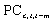is the percent change in the index for cell c from time t m to time t, in which m is equal to 1 month or 12 months previously,is the index for cell c at time t, andis the index for cell c at time t m.

### Variance estimation

Variance estimates reflect the sample distribution of the population. Basically, variance estimation answers the question, “If a survey were conducted many times, with multiple independent samples drawn from the population, what would be the spread of the sample estimates?” If the sample estimates are tightly grouped about the sample mean, the variance is small. If they are widely distributed, the variance is larger. Because conducting the survey several times is not possible, the PPI program uses a simulation method of variance estimation called bootstrapping, which involves drawing 150 replicate samples, with replacement, from the original sample and then calculating the percent change estimates for each replicate sample.

Selecting replicates:

First, within each industry, variance strata are formed, in accordance with the following criteria:

• • For probability establishments, each sampling stratum is set to be a variance stratum with each establishment serving as a primary sampling unit (PSU). If there are no additional sampling strata within an industry, then all probability establishments are placed into a single variance stratum.
• Certainty establishments with more than one item become their own variance stratum. Within this variance stratum, each item is a variance PSU.
• Certainty establishments from which only one item is collected are paired with similar units, with each item being its own variance PSU. As many as three of these units can be collapsed into a single variance stratum. If no like unit exists, this single-item unit is paired with a multi-item certainty unit to form a variance stratum. Again, each item is a variance PSU. Finally, if there are no other certainty units in the sample, this single-item certainty unit is included in every replicate.
After the variance strata are defined, 150 bootstrap replicates are selected from the sample. Each replicate is selected in the following manner:

• Of the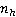PSUs in each variance stratum, a replicate sample is of size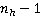. One exception is when there is a certainty unit containing a single item and there are no like establishments with which to pair that unit. In this circumstance, the unit/item is the only variance PSU in its variance stratum, and it is included in every replicate. Another exception occurs when there is only one probability unit in a sample; in that case, that unit also is included in all replicates.
• Using the sample as a frame, the PPI selects each replicate by means of random sampling with replacement. This strategy gives each PSU an equal probability of selection and allows a PSU to be selected more than once.

Reweighting:

Item weights are rescaled in each replicate with the use of the formula,

where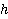is the variance stratum,is the variance PSU,is the item,is the bootstrap weight,is the weight of the original item,is the number of original sample units in the variance stratum, andis the number of times variance PSUin stratumis selected in a given replicate.

Calculating variance estimates:

Variance estimates are calculated for each percent change estimate calculated by the PPI program. The variance replicates that are selected at the detailed industry level are aggregated by using PPI aggregation structures to produce estimates for all 1-month and 12-month percent changes for each replicate. Variance estimates are then calculated with the formula,

where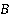is the number of bootstrap replicates (in the current implementation,= 150),is the percent change estimate for a given replicate, and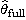is the percent change estimate of the original sample.

The standard error is the square root of the variance estimate:.

For more information, email the Producer Price Index Section of Index Analysis and Public Information at ppi-info@bls.gov, or call (202) 691-7705.